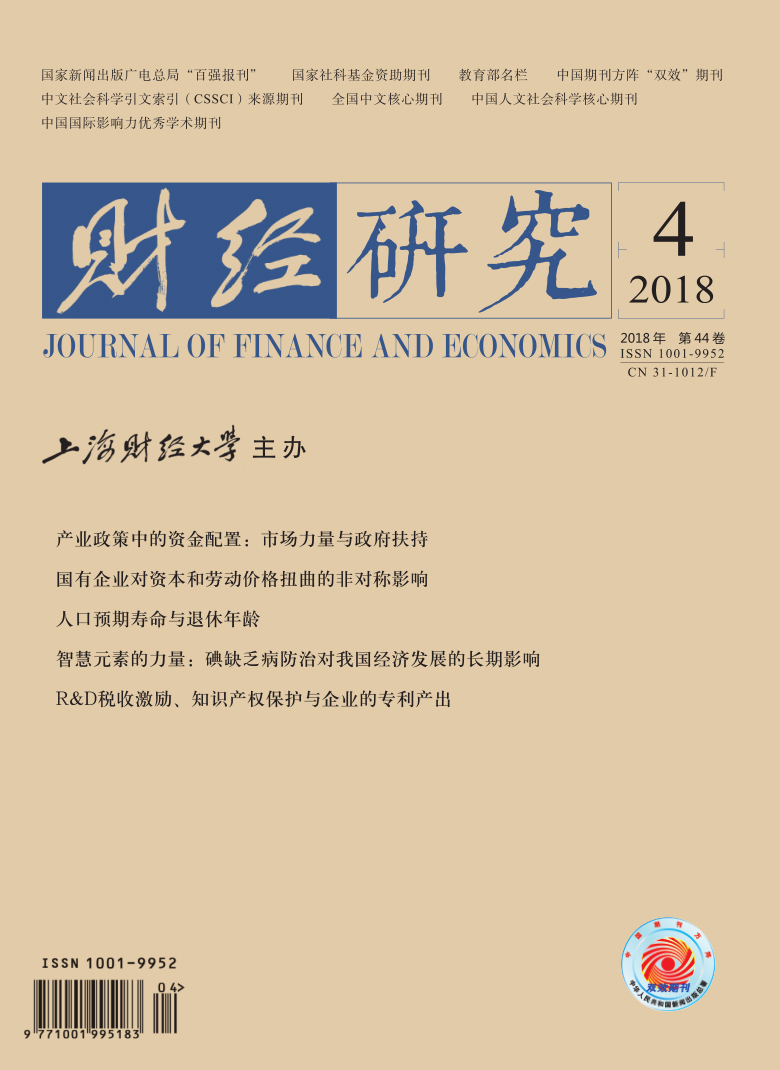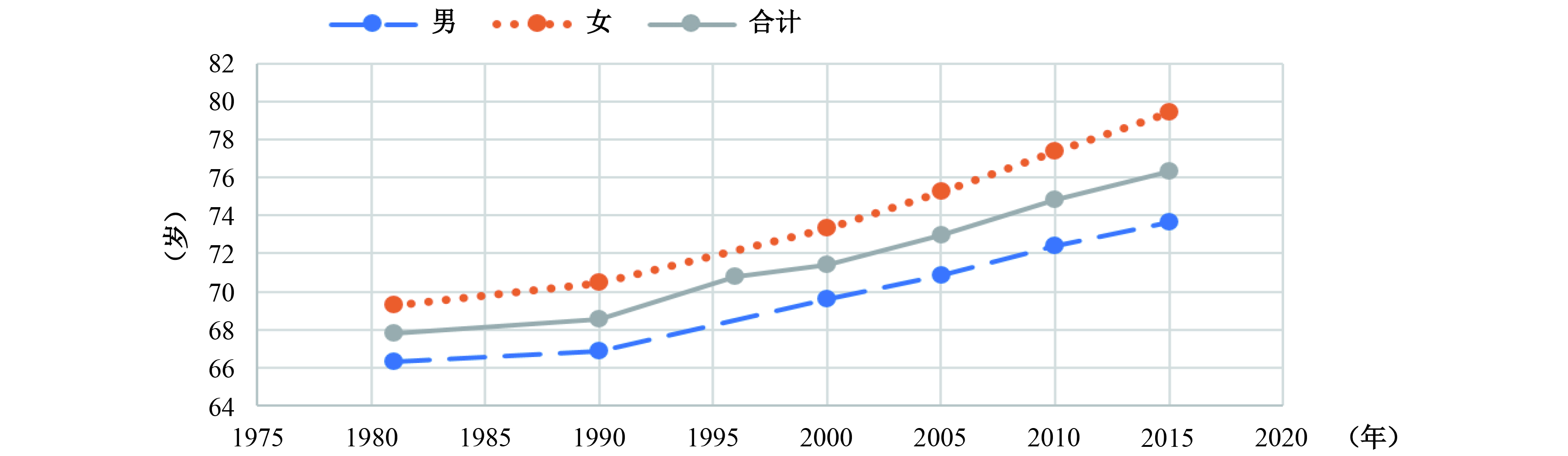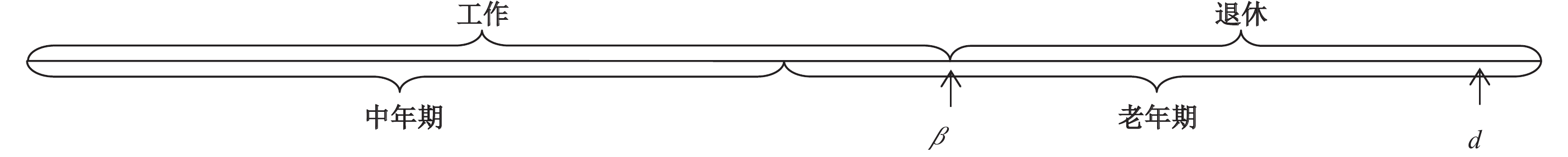﻿ 人口预期寿命与退休年龄
 财经研究2018, Vol. 44Issue (4): 62-750

#### 文章信息财经研究2018年44卷第4期

Yao Haixiang, Wei Jiahui, Ma Qinghua.

Life expectancy and retirement age

Journal of Finance and Economics, 2018, 44(4): 62-75.

### 文章历史《财经研究》
2018第44卷第4期

Life Expectancy and Retirement Age
Yao Haixiang, Wei Jiahui, Ma Qinghua
School of Finance，Guangdong University of Foreign Studies，Guangzhou 510006，China
Summary: The extension of life has exerted more and more pressure on the Chinese pension industry. In order to alleviate this pressure, more and more experts and scholars put forward policy recommendations for postponing retirement. At present, the specific reform scheme of the retirement age in China has not yet been introduced, and there are still some disputes on how to carry out the policy of postponing retirement. However, from the perspective of international experience, some countries or regions adjust retirement age directly or indirectly according to the life expectancy of population. This practice is important reference for our country. Whether China should build the adjustment mechanism of life expectancy and retirement age has very high research value. Under this background, this paper investigates the feasibility of establishing the linkage mechanism of life expectancy and retirement age from a perspective of a social planner through mathematical deduction and theoretical research, and further explores the adjustment formula between them. It hopes that this research can enrich and develop the application of overlapping generations model to the pension problem, and give relevant reference to government departments regarding the issue of postponing retirement. This paper, based on two-period overlapping generations model, introduces the retirement factors, and builds a general equilibrium framework in four aspects of individual behavior, enterprise behavior, government behavior and capital market equilibrium under the assumptions of completely competitive market, full employment and government control of the retirement age. With the optimality principle and the comparative static analysis, we find that when the economy is in a stable state, life expectancy with the maximum social welfare is positively related to the retirement age, manifesting a negative inverse proportion function form. Based on this functional relation, we use the parametric estimation method and assignment simulation to solve the adjustment formula of the life expectancy and retirement age of the population, and put forward an adjustment comparison table between them. According to the calculation results and prediction of life expectancy by China Issues National Plan on Population Development（between 2016 to 2030）, we recommend extending the retirement age to 65 years before 2030. In the end, by testing the model, on the premise of keeping the pension balance, we find that if there is no adjustment mechanism between the life expectancy and retirement age, sudden changes in life expectancy will reduce the pension payment, and can lead to the losses of social welfare. Therefore, based on the findings of this paper, and in the context of the extended life expectancy of Chinese population, we suggest that the governments should consider building the adjustment mechanism of life expectancy and retirement age, and increase the retirement age with the extension of life expectancy.
Key words: retirement age    overlapping generation model    life extension    adjustment mechanism图 1 中国人口预期寿命延长情况（1981—2015年）

 平均最低法定退休年龄/预期寿命 男性 女性 国际水平 0.8841 0.7973 中国水平 0.8148 0.6295

 解释变量 被解释变量：RAM、RAF 参数OLS估计 ${{R^2}}$ 我国RAM预测值 （基于2016年数据） 截距 斜率 LEM 43.17338***（18.15） 0.26067***（7.64） 0.2766 62.36912 LEF 45.16143***（16.04） 0.19295***（5.12） 0.1439 60.48745 注：括号中为t值，*表示p<0.1，**表示p<0.05，***表示p<0.01。
 $RAM = 43.17338 + 0.26067LEM$ (1)
 $RAF = 45.16143 + 0.19295LEM$ (2)

 ${C_{1t}} = \left( {1 - \tau } \right){w_t} - {s_t},$ (3)图 2 个人一生状态的设置

 ${C_{2t + 1}} = \beta {w_{t + 1}} + \left( {1 + {r_{t + 1}}} \right)\left( {{s_t} + {I_t}} \right) + \left( {d - \beta } \right){Q_{t + 1}},$ (4)

 ${U_t} = \ln C_{1t} + \theta d\ln {{(C_{2t + 1}/d)}}$ (5)

（一）个人行为。个人的目标是在中年、老年时期的预算约束下实现效用最大化：

 $\left\{ \begin{array}{l}{{{Max}} _{\left\{ {{s_t}} \right\}}}{U_t} = \ln C_{1t} + \theta d\ln \frac{{C_{2t + 1}}}{d},\\s.t{.}C_{1t} = \left( {1 - \tau } \right){w_t} - {s_t},\\C_{2t + 1} = \beta {w_{t + 1}} + \left( {1 + {r_{t + 1}}} \right)\left( {{s_t} + {I_t}} \right) + \left( {d - \beta } \right){Q_{t + 1}}\end{array} \right.$ (6)

 $\theta d\left( {1 + {r_{t + 1}}} \right)C_{1t} = C_{2t + 1}$ (7)

（二）企业行为。假设企业在完全竞争的环境下生产完全同质的产品，该产品可以满足所有人的消费需求，并令其价格为1。用包含技术进步的Cobb-Douglas生产函数来表示企业的生产行为：

 ${Y_t} = AK_t^\alpha {\left( {{N_t} + \beta {N_{t - 1}}} \right)^{1 - \alpha }}$ (8)

 ${\pi _t} = {Y_{{t}}} - {r_t}{K_t} - \left( {{N_t} + \beta {N_{t - 1}}} \right)\left( {1 + \eta } \right){w_t}$ (9)

 ${\pi _t} = AK_t^\alpha {\left( {{N_t} + \beta {N_{t - 1}}} \right)^{1 - \alpha }} - {r_t}{K_t} - \left( {{N_t} + \beta {N_{t - 1}}} \right)\left( {1 + \eta } \right){w_t}$ (10)

 ${r_t} = \alpha A{M^{1 - \alpha }}k_t^{\alpha - 1}$ (11)
 ${w_t} = {{\left( {1 - \alpha } \right)A{M^{ - \alpha }}k_t^\alpha }}/{{(1 + \eta )}}$ (12)

（三）政府行为。在模型中，政府部门的作用是管理养老金的缴纳和发放。按照目前我国养老保险政策有关规定，城镇居民按一定的工资比例缴纳个人账户养老金，企业按照一定的工资比例缴纳公共账户养老金。个人账户养老金由个人在工作时缴纳，退休后再发放。而公共养老金是“现给现付”的，由当期的中年人和还在参加工作的老年人缴纳给当期已经退休的老年人。

 ${I_t} = \tau {w_t}$ (13)

 $\left( {d - \beta } \right){N_t}{Q_{t + 1}} = \left( {{N_{t + 1}} + \beta {N_t}} \right)\eta {w_{t + 1}}$ (14)

 ${Q_{t + 1}} = {{\left( {1 + n + \beta } \right)\eta {w_{t + 1}}}}/{{(d - \beta )}}$ (15)

${Q_{t + 1}}$ 关于人均寿命 $d$ 求导，可以得到：

 ${{\partial {Q_{t + 1}}}}/{{\partial d}} = - {{\left( {1 + n + \beta } \right)\eta {w_{t + 1}}}}/{{{{\left( {d - \beta } \right)}^2}}} < 0$ (16)

（四）资本市场。Blanchard和Fischer（1988）具体推导了资本市场均衡的条件。假设资本完全折旧，资本市场均衡要求上一期的总储蓄构成了下一期期初的资本存量，由此可以得出市场均衡条件：

 ${N_{t + 1}}{k_{t + 1}} = {N_t}\left( {{s_t} + {I_t}} \right)$ (17)

 ${s_t} = \left( {1 + n} \right){k_{t + 1}} - {I_t}$ (18)

 ${s_t} = \left( {1 + n} \right){k_{t + 1}} - {{\tau \left( {1 - \alpha } \right)A{M^{ - \alpha }}k_t^\alpha }}/{{(1 + \eta )}}$ (19)

（五）动态均衡。由式（11）−（13）、式（15）和式（19），可分别得到利率 ${r_{t + 1}}$ 、中年工资 ${w_t}$ 、老年工资 ${w_{t + 1}}$ 、个人账户养老金 ${I_t}$ 、储蓄 ${s_t}$ 、公共账户养老金 ${Q_{t + 1}}$ 关于人均资本 ${k_{t + 1}}$ ${k_t}$ 的解析式。把式（11）−（13）、式（15）和式（19）同时代入一阶必要条件式（7）中，可得到关于人均资本 ${k_{t + 1}}$ ${k_t}$ 的差分方程：

 $\begin{array}{l}\theta d\left( {1 + \alpha A{M^{1 - \alpha }}k_{t + 1}^{\alpha - 1}} \right)\left[ {\displaystyle\frac{{\left( {1 - \alpha } \right)A{M^{ - \alpha }}k_t^\alpha }}{{1 + \eta }} - \left( {1 + n} \right){k_{t + 1}}} \right] - \displaystyle\frac{{\beta \left( {1 - \alpha } \right)A{M^{ - \alpha }}k_{t + 1}^\alpha }}{{1 + \eta }}\\[12pt] - \left( {1 + \alpha A{M^{1 - \alpha }}k_{t + 1}^{\alpha - 1}} \right)\left( {1 + n} \right){k_{t + 1}} - \displaystyle\frac{{\left( {1 + n + \beta } \right)\eta \left( {1 - \alpha } \right)A{M^{ - \alpha }}k_{t{{ + }}1}^\alpha }}{{1 + \eta }} = 0\end{array}$ (20)

（六）社会福利最大化。政府部门与个人、企业部门不同，是非盈利的机构。除了管理养老金的缴纳和发放外，政府也是养老保险政策的制定者，可以决定退休年龄，也就是常说的社会计划者（Social Planner）。从社会计划者角度出发，政府的工作是实现社会福利的最大化。取单个人的一生中效用函数作为代表性个体的福利函数，把从第0代开始到第 $i$ 代人的福利函数进行加总，加总后得到的为社会福利函数，并令其为 $V$ ，那么有：

 $V = \theta d\ln \frac{{C_{20}}}{d} + \sum\limits_{i = 0}^\infty {{\xi ^i}\left[ {\ln C_{1i} + \theta d\ln \frac{{C_{2i + 1}}}{d}} \right]}$ (21)

 ${N_i}{k_i} + {Y_i} = {N_{i + 1}}{k_{i + 1}} + {N_i}C_{1i} + {N_{i - 1}}C_{2i}$ (22)

 ${k_i} + A{M^{1 - \alpha }}k_i^\alpha = \left( {1 + n} \right){k_{i + 1}} + C_{1i} + {{C_{2i}}}/{{(1 + n)}}$ (23)

 $k = {\left[ {{{(1 + n - \xi) }}/{{\alpha A\xi }}} \right]^{\frac{1}{{\alpha - 1}}}}M$ (24)

 $\begin{array}{l}\theta d\left( {1 + \alpha A{M^{1 - \alpha }}k^{\alpha - 1}} \right)\left[ {\displaystyle\frac{{\left( {1 - \alpha } \right)A{M^{ - \alpha }}k^{\alpha - 1}}}{{1 + \eta }} - \left( {1 + n} \right)} \right] - \displaystyle\frac{{\beta \left( {1 - \alpha } \right)A{M^{ - \alpha }}k^{\alpha - 1}}}{{1 + \eta }}\\[10pt] - \left( {1 + \alpha A{M^{1 - \alpha }}k^{\alpha - 1}} \right)\left( {1 + n} \right) - \displaystyle\frac{{\left( {1 + n + \beta } \right)\eta \left( {1 - \alpha } \right)A{M^{ - \alpha }}k^{\alpha - 1}}}{{1 + \eta }} = 0\end{array}$ (25)

 $\beta = \frac{{\theta d\left( {1 + n} \right)\left[ {\left( {1 - \alpha } \right)\left( {1 + n - \xi } \right) - \alpha \xi \left( {1 + \eta } \right)\left( {1 + n} \right)} \right] - \eta \xi \left( {1 - \alpha } \right)\left( {1 + n - \xi } \right)\left( {1 + n} \right) - \alpha \xi \left( {1 + \eta } \right){{\left( {1 + n} \right)}^2}}}{{\xi \left( {1 + \eta } \right)\left[ {\alpha \left( {1 + n} \right)\left( {1 + \theta d} \right) + \left( {1 - \alpha } \right)\left( {1 + n - \xi } \right)} \right]}}$ (26)

（一）稳态下的利率、工资与最优退休年龄。将稳定状态下的人均资本式（24）分别代入到利率式（11）与工资式（12）中，可以得到稳定状态下的利率和工资：

 $r = {{(1 + n - \xi )}}/{\xi }$ (27)
 $w = {{\left( {1 - \alpha } \right)A}}/{{(1 + \eta) }}{\left[ {{{(1 + n - \xi )}}/{{\alpha A\xi }}} \right]^{\frac{\alpha }{{\alpha - 1}}}}$ (28)

 ${{\partial {w_t}}}/{{\partial \beta }} = - {{\left( {1 - \alpha } \right)\alpha A{M^{ - \alpha - 1}}k_t^\alpha }}/{{\left( {1 + \eta } \right)\left( {1 + n} \right)}} < 0$ (29)

 ${{\partial k}}/{{\partial \beta }} = {\left[ {{{(1 + n - \xi )}}/{{\alpha A\xi }}} \right]^{\frac{1}{{\alpha - 1}}}}{{(2 + n)}}/{{(1 + n)}} > 0$ (30)

 ${{\partial {r_t}}}/{{\partial \beta }} = {{\alpha \left( {1 - \alpha } \right)A{M^{ - \alpha }}k_t^{\alpha - 1}}}/{{(1 + n)}} > 0$ (31)

（二）人口预期寿命最优退休年龄。化简式（26）的稳态下的最优退休年龄，可得以下函数：

 $\beta = {({e}/{g})} - {{\left[({eh}/{g}) - f\right]}}/{{(gd + h)}}$ (32)

 \left\{ \begin{aligned}& e = \theta \left( {1 + n} \right)\left[ {\left( {1 - \alpha } \right)\left( {1 + n - \xi } \right) - \alpha \xi \left( {1 + n} \right)\left( {1 + \eta } \right)} \right]\\& f = - \eta \xi \left( {1 - \alpha } \right)\left( {1 + n - \xi } \right)\left( {1 + n} \right) - \alpha \xi \left( {1 + \eta } \right){\left( {1 + n} \right)^2}\\& g = \alpha \theta \xi \left( {1 + n} \right)\left( {1 + \eta } \right)\\& h = \xi \left( {1 + \eta } \right)\left[ {\left( {1 + n} \right) - \left( {1 - \alpha } \right)\xi } \right]\end{aligned} \right. (33)

 ${{\partial \beta }}/{{\partial d}} = {{(eh - gf)}}/{{{{\left( {gd + h} \right)}^2}}} > 0$ (34)

 ${{{\partial ^2}\beta }}/{{\partial {d^2}}} = - {{(egh - {g^2}f)}}/{{{{\left( {gd + h} \right)}^4}}} < 0$ (35)图 3 人口预期寿命和退休年龄的调整机制

（一）参数估计。

1. 人口预期寿命 $d$ 、公共和个人养老金缴费率 $\tau$ $\eta$ 、人口增长率 $n$ 和技术进步 $A$ 。公共养老金缴费率和工人养老金缴费率可以按照政府相关规定设置。根据《国务院关于完善企业职工基本养老保险制度的决定》中的规定，可以得到公共缴费率 $\eta = 0.2$ ，个人缴费率 $\tau = 0.08$ 。而从2016年中国统计年鉴中可以得到人口预期寿命和人口增长率的赋值：以男性为例，2015年的人均预期寿命为73.64岁，转化到模型中有 $d = {{(73.64 - 50)}}/{{30}} = 0.788$ ；对于人口增长率而言，目前中国人口增速渐缓，并且在2015年10月首次提出了放开二胎的生育政策。现假设2015年的人口自然增长率0.496%为稳态下的人口增长率，于是有 $n = {(1.00496)^{30}} - 1 =$ 0.1600。本模型着重考察经济处于稳定状态下的退休年龄和人口预期寿命的关系，由式（26）可知，技术进步并不会对结论造成影响。现假设经济处于稳定状态下的全要素生产率不会发生改变，并令 $A = 1$

2. 代际折现率 $\xi$ 。代际折现率在本模型中表示的是未来代的消费效用对社会计划者的重要程度。马贤磊和曲福田（2011）认为，代际折现率应该从道德和经验两个角度考察，存在一定的量化困难。在本模型中，可以通过稳态水平下的利率找到代际折现率。从2015−22017年，我国的银行5年定期存款基准利率为2.75%，由于现实经济社会中社会的投资渠道更为丰富，收益率更高。林忠晶（2007）认为，交叠世代模型中利率在8%到11%之间比较合适。经过权衡，假设经济处于稳态下的年利率为8%，通过计算，可以得到利率 $r = \displaystyle\frac{1}{{30}}\sum\limits_{i = 1}^{30} {{{\left( {1{{ + }}0.08} \right)}^i}} {{ = }}4.0782$ 并将其代入至式（27）中，可以得到代际折现率 $\xi = 0.2284$

3. 资本收入份额 $\alpha$ 。白重恩和钱震杰（2009）在研究资本收入份额的影响因素时，使用除劳动力报酬以外的其他因素占GDP的份额来测算资本收入份额。通过2016年中国统计年鉴，可以测算得到2015年全国资本收入份额大概为0.5左右。由于本模型存在资本完全折旧和充分就业的假设，模型中资本收入份额可能会比实际更低。康传坤（2014）、杨再贵（2008）将资本收入份额设置为0.35，参照前人的做法，本文也将资产收入份额定为 $\alpha = 0.35$

4. 效用折现率 $\theta$ 。效用折现率衡量老年时期消费得到的效用对于中年期的重要程度，具有一定的量化难度。顾六宝和肖红叶（2004）根据凯恩斯-拉姆齐模型给出了两种效用折现率的推算方法，用以衡量人们每年对风险偏好心态的变化轨迹。而本模型中的效用折现率时间跨度较大，希望能寻找到一个比较稳定的效用折现率来反映老年消费生活对中年的影响。目前来讲，对是否要延迟退休还存在相当大的争议，不妨以男性为例，假设目前法定退休年龄为最优，以考察未来人口预期寿命发生变化时退休年龄应如何调整。通过代入参数至式（26）可以计算出效用折现率为 $\theta = 0.6001$

（二）敏感性分析。以上参数中，资本收入份额和效用折现率难以准确测算，有必要分别进行敏感性分析。

1. 对参数资本收入份额 $\alpha$ ，分别设置0.25、0.35和0.45，可以得到：

 参数设置 最优退休年龄 ${d = 0.788}$ 、 ${n = 0.1600}$ 、 ${\xi = 0.2284}$ 、 ${\alpha = 0.30}$ 、 ${\theta = 0.6001}$ ${\beta = 0.5018}$ （65.06岁） ${d = 0.788}$ 、 ${n = 0.1600}$ 、 ${\xi = 0.2284}$ 、 ${\alpha = 0.35}$ 、 ${\theta = 0.6001}$ ${\beta = 0.3333}$ （60.00岁） ${d = 0.788}$ 、 ${n = 0.1600}$ 、 ${\xi = 0.2284}$ 、 ${\alpha = 0.40}$ 、 ${\theta = 0.6001}$ ${\beta = 0.1754}$ （55.26岁）

2. 对参数效用折现率 $\theta$ ，分别设置0.5001和0.6001和0.7001，可以得到：

 参数设置 最优退休年龄 ${d = 0.788}$ 、 ${n = 0.1600}$ 、 ${\xi = 0.2284}$ 、 ${\alpha = 0.35}$ 、 ${\theta = 0.5001}$ ${\beta = 0.2017}$ （56.05岁） ${d = 0.788}$ 、 ${n = 0.1600}$ 、 ${\xi = 0.2284}$ 、 $\alpha = 0.35$ 、 ${\theta = 0.6001}$ ${\beta = 0.3333}$ （60.00岁） ${d = 0.788}$ 、 ${n = 0.1600}$ 、 ${\xi = 0.2284}$ 、 ${\alpha = 0.35}$ 、 ${\theta = 0.7001}$ ${\beta = 0.4581}$ （63.74岁）

（三）人口预期寿命和退休年龄调整机制。基于模型的求解和参数估计，下面给出人口预期寿命和退休年龄的调整公式，以供政府参考。基于以上参数估计，得到以下参数估计表：

 ${\alpha}$ ${\theta}$ ${\eta}$ ${\tau}$ ${n}$ ${\xi}$ 0.35 0.6001 0.2 0.08 0.1600 0.2284

 $RA = 204.5733 - {{47.6894}}/{{(0.0668LE + 4.9784)}}$ (36)

 预期寿命 退休年龄 预期寿命 退休年龄 预期寿命 退休年龄 预期寿命 退休年龄 68.69 55 72.62 59 76.78 63 81.18 67 69.65 56 73.64 60 77.86 64 82.32 68 70.63 57 74.67 61 78.95 65 83.48 69 71.62 58 75.72 62 80.06 66 84.65 70

《国家人口发展规划（2016−2030年）》预测中国2030年人口预期寿命达到79岁。根据本模型的结论，建议在2030年前根据寿命的延长逐步延迟退休年龄至65岁。

 调整退休年龄 ${d_i}$ ${\beta _i}$ ${Q_i}$ ${C_{2i}}$ ${{{{C_{2i}}}}/{{{d_i}}}}$ ${U_t}$ ${i = t}$ 0.7880 0.3333 0.0948 0.2647 0.3359 −2.8958 ${i = \left( {t + 1} \right)}$ 0.9667 0.5016 0.1032 0.2939 0.3040 不调整退休年龄 ${d_i}$ ${\beta _i^b}$ ${Q_i^b}$ ${C_{2i}^b}$ ${{{C_{2i}^b}}/{{d_i^b}}}$ ${{U_t^b}}$ ${i = t}$ 0.7880 0.3333 0.0948 0.2647 0.3359 −2.9565 ${i = \left( {t + 1} \right)}$ 0.9667 0.3333 0.0681 0.2647 0.2738

① 限于篇幅，这里不贴出具体数据。可以自行查阅世界银行数据库http://web.worldbank.org，其中各国法定退休年龄数据更新至2013年。

② 本文采用了退休年龄完全由政府控制的外生方式建模，而实际上政府能够决定的仅为领取退休金的法定退休年龄，而非实际的退休年龄。这在一定程度上不符合实际情况。但相信现实生活中，法定退休年龄是具有一定的指导意义的，这让大部分人最终选择在法定的退休年龄退休。

③ 按照假设，60岁退休可以计算出β=（60−50）/30=0.3333，73.64岁的人口预期寿命可以计算出d=（73.64−50）/30=0.788。

④ 在老年时期，缴纳的个人账户养老金会在退休后全部发回。按照模型假设，延迟退休参加工作与实际退休同属一个时期，不存在利率的回报，所以这里提前把个人账户养老金的缴纳和发放相抵消了。

⑤ 具体求解过程见附录。

⑥ 稳态条件求解过程略，但备索。

⑦ 其中i趋向于无穷。

⑧ 社会福利最大化求解过程略，但备索。

⑨ 详细证明过程略，但备索。

⑩ 模型中设置的利率r为一个时期所有储蓄的回报。假设个人每年做同样的储蓄，可以通过等比数列求和的方法计算利率r

  白重恩, 钱震杰. 我国资本收入份额影响因素及变化原因分析——基于省际面板数据的研究[J]. 清华大学学报（哲学社会科学版）, 2009(4): 137–147.  胡仕强, 许谨良. 长寿风险、养老金体制与资本积累[J]. 财经研究, 2011(8): 125–134.  黄健元, 石晶晶. 基于BP算法的人口平均寿命预测[J]. 统计与决策, 2007(6): 60–62.  康传坤. 人口老龄化背景下养老保险与退休年龄关系的研究[D]. 成都: 西南财经大学, 2014.  乐菡. 基于三期OLG模型的老龄社会城镇职工基本养老保险发放水平影响因素研究——以上海市为例[J]. 老龄科学研究, 2016(1): 20–33.  李志生, 吕勇斌, 刘恒甲. 长寿风险的识别与量化研究: 来自中国的数据[J]. 统计与决策, 2011(16): 72–74.  连祥卿. 人口老龄化对劳动年龄人口的影响[J]. 同济医科大学学报, 1990(1): 55–58.  林宝. 延迟退休年龄对养老金资金平衡的影响[J]. 财经问题研究, 2014(12): 41–46. DOI:10.3969/j.issn.1000-176X.2014.12.007  林忠晶, 龚六堂. 退休年龄、教育年限与社会保障[J]. 经济学（季刊）, 2007(1): 211–230.  马贤磊, 曲福田. 成本效益分析与代际公平: 新代际折现思路[J]. 中国人口•资源与环境, 2011(8): 22–28.  王晓军, 赵明. 寿命延长与延迟退休: 国际比较与我国实证[J]. 数量经济技术经济研究, 2015(3): 111–128.  魏翔. 基于闲暇时间−效用函数的居民消费研究——对中国数据的实证检验[J]. 经济科学, 2006(4): 104–113.  谢世清. 长寿风险的创新解决方案[J]. 保险研究, 2011(4): 70–75.  许伟, 陈斌开. 银行信贷与中国经济波动: 1993−2005[J]. 经济学（季刊）, 2009(3): 969–994.  阳义南, 曾燕, 瞿婷婷. 推迟退休会减少职工个人的养老金财富吗?[J]. 金融研究, 2014(1): 58–70.  杨再贵. 企业职工基本养老保险、养老金替代率和人口增长率[J]. 统计研究, 2008(5): 38–42.  殷俊, 黄蓉. 人口老龄化、退休年龄与基础养老金长期偿付能力研究[J]. 理论与改革, 2012(4): 73–76.  曾燕, 郭延峰, 张玲. 基于长寿风险与OLG模型的延迟退休决策[J]. 金融经济学研究, 2013(4): 83–93.  张熠. 延迟退休年龄与养老保险收支余额: 作用机制及政策效应[J]. 财经研究, 2011(7): 4–16.  Aubert P, Duc C, Ducoudré B. French retirement reforms and intragenerational equity in retirement duration[J]. De Economist, 2013, 161(3): 277–305. DOI:10.1007/s10645-013-9212-6  Casamatta G, Cremer H, Pestieau P. The political economy of social security[J]. Scandinavian Journal of Economics, 2000, 102(3): 503–522. DOI:10.1111/sjoe.2000.102.issue-3  Casamatta G, De Paoli C. Choosing the legal retirement age in presence of unemployment[J]. Recherches Économiques de Louvain / Louvain Economic Review, 2012, 78(1): 5–25. DOI:10.3917/rel.781.0005  Chai J J, Horneff W, Maurer R. Optimal portfolio choice over the life cycle with flexible work, endogenous retirement, and lifetime payouts[J]. Review of Finance, 2011, 15(4): 875–907. DOI:10.1093/rof/rfr016  Hugonnier J, Pelgrin F, St-Amour P. Health and （other） asset holdings[J]. The Review of Economic Studies, 2013, 80(2): 663–710. DOI:10.1093/restud/rds033  Kalwij A, Kapteyn A, De Vos K. Retirement of older workers and employment of the young[J]. De Economist, 2010, 158(4): 341–359. DOI:10.1007/s10645-010-9148-z  Lacomba J A, Lagos F. Population aging and legal retirement age[J]. Journal of Population Economics, 2006, 19(3): 507–519. DOI:10.1007/s00148-005-0044-9  Michello F A, Ford W F. The unemployment effects of proposed changes in social security’s " normal retirement age”[J]. Business Economics, 2006, 41(2): 38–46. DOI:10.2145/20060205  Pecchenino R A, Pollard P S. Dependent children and aged parents: Funding education and social security in an aging economy[J]. Journal of Macroeconomics, 2002, 24(2): 145–169. DOI:10.1016/S0164-0704(02)00024-1  Weller C E. Raising the retirement age for social security: Implications for low wage, minority, and female workers[R]. Working Paper: Center for American Progress, Washington, DC, 2005.  Zhang J S, Zhang J, Lee R. Mortality decline and long-run economic growth[J]. Journal of Public Economics, 2001, 80(3): 485–507. DOI:10.1016/S0047-2727(00)00122-5